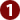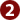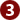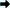STUDY
AND
EXAM
.COM

# NTS GAT General Test – reparation Guidelines

Graduate Assessment Test (GAT) General is a test designed by NTS for assessment of candidate for admission in MS/MPhil, scholarships and recruitments.

GAT General Test consists of following three parts:

1. Verbal reasoning
2. Quantitative reasoning
3. Analytical reasoning

The proportion of each part vary in test depending on purpose of test (i.e. admission, scholarship, recruitment etc) or discipline of admission if it is attempted for seeking admission in MS/MPhil.## Preparation for Verbal reasoning (English)

This part is designed to assess your grammatical knowledge and vocabulary with regards to its correct usage. The questions include:

1. Correct usage of verb, adverb, conjunction and prepositions in sentences
2. Synonyms and Antonyms
3. Analogies
4. Tense related questions
5. Questions on paragraph, title and words to relate in closest meaning

Guidelines for preparation are as follows:

1. Learn the basics of English tenses because most of the questions are on usage of correct form of verb and auxiliaries. Example: I have ____ a poem. (A) write (B) wrote (C) written. Answer: I have written a poem.
2. Learn correct usage of some common prepositions. Every test has atleast two or more MCQs about correct usage of preposition. Example: Don’t laugh ___ poor man. (A) on (B) about (C) at (D) over. Answer: Don’t laugh at poor man.
3. Study MCQs books of high level (i.e. SAT-I). Most of ‘synonyms, antonyms and analogies questions’ are taken from such books for MCQs tests. It will cover many of your question as those question are comparatively more in number in the test.
4. You should study standard books of English grammar which discusses the grammar concepts in details.
5. Study some good locally available books of MCQs. These provide you with a huge bank of MCQs which can be easily prepared in a short time.## Preparation for Quantitative reasoning (Mathematics)

The quantitative reasoning includes simple arithmetic questions. These are easy questions but involve thought provoking tricks. The questions include:

1. Arithmetics question i.e. addition, subtraction, division and multiplication
2. Percentages
3. Ratio and Proportion
4. Simple Algebra
5. Simple geometry i.e. area of shapes and volumes of bodies.

Guidelines for preparation are as follows:

1. These are very easy question because these are designed for all students coming from different educational disciplines. Questions are based on simple arithmetics of class eight, nine and ten.
2. Learn the short formulas to solve questions quickly. Example: What is sum of number from 1 to 100? If you start adding all the numbers from 1 to 100, it will take hours. But it has a simple formula.
3. Sum of digits = number of digits (first digit + last digit)/2
Sum of digits = 100(1 +100)/2 = 5050 Answer

4. For geometry, learn the basic formulas of area of shapes and volumes of bodies. Sometimes you may be asked to find area of an unusual shape. Remember, such shapes are basically a combination of squares and triangle. You have to find area of individual square and triangle. Add areas of all squares and triangles (as part of the shape) to calculate total area of the unusual shape. If a semi-circle is also a part of the same shape. Find area of circle and divide by two.
5. Learn Pythagorean theorem conceptually. It is favorite area of test makers. Pythagorean theorem is used to find length of one side of a triangle. You may be asked to find a length between two points in a scenario. It may be basically a triangle, where you need to use Pythagorean theorem. Example: A common question where length of wall and floor is given and you are asked to find length of ladder to reach to top of wall.
6. Learn the basics of calculating percentages as well as ratios.
7. Questions are easy but involve simple arithmetic tricks. See the following examples:

Question: If ¾ of a number is 7 more than 1/6 of the number, what is the number?
Options:  A. 12  B. 15    C. 18   D. 20## Preparation for Analytical reasoning:

Analytical reasoning part is designed to assess your analytical skills, mental sharpness and situational processing skills. You are given certain facts and rules. You are asked to process the facts by applying rules.

For example: Five educational documentaries, namely A, B, C, D and E, are to be shown to students in the following manners.

1. A must be shown earlier than D
2. B must be shown earlier than D
3. E must be shown on fifth number

Q. No. 1. Which can be the correct order of showing documentaries?
(A)  A, C, B, D, E                 (B)  A, C, D, E, B
(C)  B, D, C, A, E                 (D)  B, D, C, A, E

Q. No. 2. If C is shown earlier than E, which of the following stand true?
(A) A is the first film shown     (B) B is the second film shown
(C) C is the third film shown    (D) D is the fifth film shown

Guidelines are as follows:

1. During the test, be attentive and alert mined.
2. While attempting the question, focus on both facts and rules at same time. Have both of them retained in your mind. Usually students focus only on the facts ignoring the rules or vice versa.
3. If rule statement are too long. Better make a short symbols or formula. It will help you easily grasp them while focusing on facts and the given options(answers).
4. Mostly you are asked about making combination of items with rules. Suppose, different groups of three alphabets each are to be made from given 6 alphabets, i.e. A, B, C, D, E and F. Rules are given that in a same group B and E cannot come together; and a group having F must have C too. Better practice such questions.
5. Practice such test from books in order to learn the nature of such questions and tactics to solve them.

## Test Taking Strategies

1. Time for MCQs test is usually short. Divide the total time on all MCQs to get for time for each MCQ.
2. Have a wrist watch. Keep an eye on it to move on speedily while attempting MCQs.
3. Read every MCQ carefully as well as speedily to complete the test in time.
4. Attempt all the easy MCQs first and leave the difficult MCQs for the end. Encircle the number of each un-attempted MCQ to identify it easily in the end.
5. Do not stick to one MCQ if it is taking time. It will waste your time for other easy MCQs.
6. If you realize that you cannot answer a MCQ because you don’t have any idea about it. First skip out those options about which you are sure that these cannot be the answer. Selecting from the remaining option maximize chance of selecting the correct option.
7. Practice sample tests while making preparation for the real test. You should learn the content but you should also learn tactics of attempting MCQ test. You can have many sample test, practice them at home just like taking your real test. You will learn completing the test in time as well as how to attempt MCQs in correct way.Click to attempt 'NTS Practice Test'